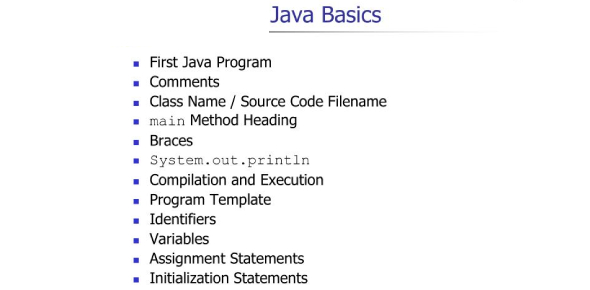# Java Basics: Trivia Questions Quiz! Exam

9 Questions | Total Attempts: 34Settings.

• 1.
By convention, method names begin with an uppercase first letter, and all subsequent words in the name begin with a capital first letter.
• A.

True

• B.

False

• 2.
Any class that contains public static void main (Strings [] args) can be used to execute an application.
• A.

True

• B.

False

• 3.
A set of statements contained within a pair parenthesis is called a block.
• A.

True

• B.

False

• 4.
If an increment operator is ___________ to a variable, first the variable is incremented by 1, then its new value is used in the expression.
• 5.
Instance variable of type  char, byte, short, int, long and double are all give the value ______ by default.
• 6.
The _____ statement selects among multiple actions based on the possible values of an integer variable or expression.
• 7.
The expression ((x>y) && (a<b)) is true if either (x>y) is true or (a<b) is true.
• A.

True

• B.

False

• 8.
List and tables or values can be stored in ________.
• 9.
The number used to refer to a particular element of an array is called the element's __________.
Related TopicsBack to top